maths.org Go back Open original

# Unveiling the Mandelbrot set

x0 = 0,
x1 = -1.01,
x2 = 0.0101,
x3 = -1.00989799,
x4 = 0.00989395,
x5 = -1.00990210,
x6 = 0.00990227,
and so forth. The points in the orbit get closer and closer to the 2-cycle, without ever landing right on it — mathematicians say that the orbit of zero is attracted to the two cycle.

This is the case not only for c=-1.01: for any c value that lies inside the same bulb as -1.01 the function x2 + c has a 2-cycle and the orbit of zero is attracted to it.

Something similar happens for every primary bulb: if c lies in the interior of such a primary bulb, then the orbit of 0 is attracted to a cycle of some period n. The number n is the same for any c inside this primary bulb, and it is called the period of the bulb. To see that this is really true, open the Mandelbrot set iterator applet on my website, which was written by James Denvir. Clicking on a point in the Mandelbrot set shown in this applet will display the orbit of zero on the left. You will see that after a few iterations this orbit cycles.

Using the applet you can verify that the periods of some of the larger primary bulbs are as shown in figure 7. Simply click on a c-value inside a bulb and count the number of points in the cycle.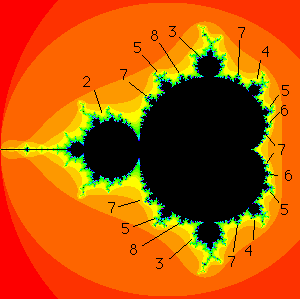Figure 7: the periods of the bulbs

Figure 8 shows close-ups of the period 3, 4, 5 and 7 bulbs (in clockwise order starting from the top left). Now count the spokes of the largest antenna attached to each bulb (don't forget to count the spoke emanating from the primary bulb to the main junction point of the antenna.) What we are beginning to see here is a remarkable relationship: the number of spokes is precisely the period of the primary bulb!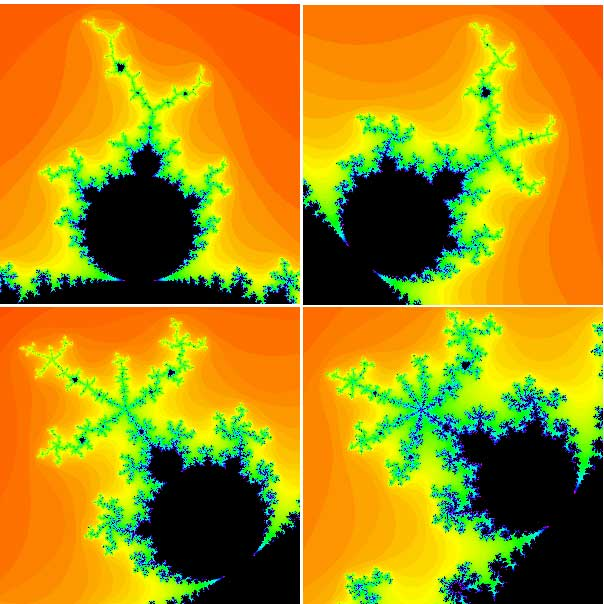Figure 8: various bulbs and their periods. Clockwise from top left: period 3 bulb, period 4 bulb, period 5 bulb, period 7 bulb. Note that the period of the bulb is equal to the number of spokes on the main antenna.

A similar result is true for non-primary bulbs that are not directly attached to the main cardioid. In this case, the period of the bulb is a multiple of the number of spokes attached to its antenna.

### Filled Julia sets

But the remarkable relationships don't end here. There is a second, more dynamic way to calculate the periods of these primary bulbs in M. To explain this, we have to introduce the notion of a filled Julia set. The filled Julia set for x2 + c is subtly different from the Mandelbrot set. For M, we calculated only the orbit of 0 for each c-value and then displayed the result. A c-value lies in M if the corresponding orbit of 0 does not escape to infinity. The Mandelbrot set is a picture in the c-plane.

For the filled Julia sets, we fix a c-value and then consider the fate of all possible seeds for that fixed value of c. Those seeds whose orbits do not escape form the filled Julia set of x2 + c. Putting it formally:

Fix a value of c. The filled Julia set of x2 + c is the collection of seeds whose orbit does not tend to infinity.

(Caution: There is also a notion of the Julia set. This term is used to describe the boundary of the filled Julia set.)

From our examples above we see that the point 0 lies in the filled Julia set of x2 + 0, because it is fixed and does not go anywhere. But 0 is not in the filled Julia set of x2 + 1, because it escapes to infinity.

Thus, we get a different filled Julia set for each different choice of c. We write Jc for the filled Julia set of x2 + c. In figures 9 to 12 we have displayed the filled Julia sets for a variety of c-values.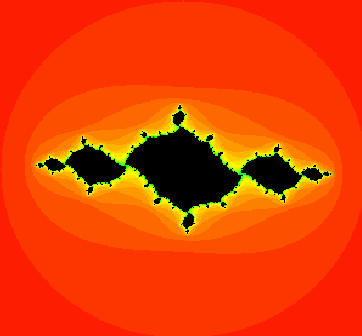Figure 9: the filled Julia set for c = -1.037 + 0.17i, which lies in a period 2 bulb.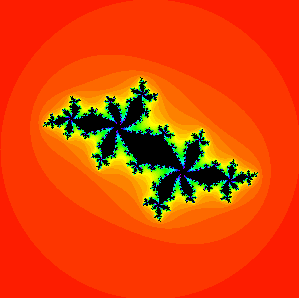Figure 10: the filled Julia set for c = -0.52 + 0.57i, which lies in a period 5 bulb.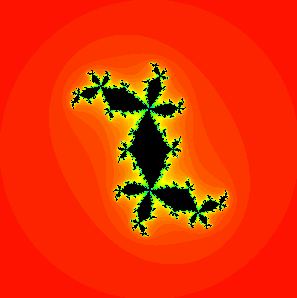Figure 11: the filled Julia set for c = 0.295 + 0.55i, which lies in a period 4 bulb.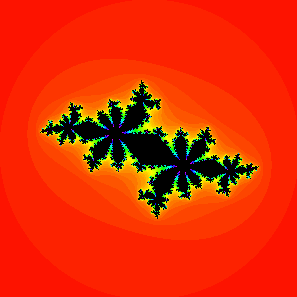Figure 12: the filled Julia set for c = -0.624 + 0.435i, which lies in a period 7 bulb.

To get a feel for filled Julia sets, see if you can show that the filled Julia set for c = 0 is a round disc. Most filled Julia sets, however, are impossible to work out without a computer. To see some of them open the Mandelbrot and Julia set applet on my website, which was written by Yakov Shapiro. Clicking on a point in the window with the Mandelbrot set on the right specifies a c-value, and then clicking "compute" will display the corresponding filled Julia set on the left.

To summarise, here is the difference between Mandelbrot and Julia sets again:

The Mandelbrot set

• is a picture in the c-plane, also called the parameter plane,
• records the fate of the orbit of 0 for all possible c-values.

The filled Julia set

• is a picture in the x-plane, also called the dynamical plane,
• records the fate of all orbits for x2 + c for a fixed c. There is one Julia set for each value of c.

### The fundamental dichotomy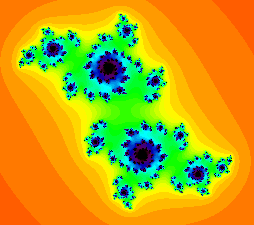This Julia set is a Cantor set. It is made up of the black points. Every such point forms a separate component, and these point components pile up on each other.

All the filled Julia sets we have displayed here have one thing in common: they are connected sets, in other words they consist of just one piece. But not all filled Julia sets are connected. One of the most beautiful results in all of complex dynamics dates back to 1919 and was proved independently by both Gaston Julia and Pierre Fatou. It states that either a Julia set is connected, or it consists of infinitely many pieces, each of which is a single point. These points pile up on each other creating something resembling a cloud. "Cloud sets" like these are called Cantor sets (see Plus article How big is the milky way? for more on Cantor sets).

So the fundamental dichotomy says that filled Julia sets for x2 + c come in one of two varieties: connected sets (one piece) or Cantor sets (infinitely many pieces). There is no in-between: there are no c-values for which Jc consists of 10 or 20 or 756 pieces.

How do we decide what shape a given Jc assumes, whether it is connected or a Cantor set? Amazingly, it is the orbit of 0 that determines this. For if the orbit of 0 tends to infinity under iteration of x2 + c, then the fact is that Jc is a Cantor set. On the other hand, if the orbit of 0 does not tend to infinity, then Jc is a connected set.

A visual way to view this dichotomy is given by the Mandelbrot set. If c lies in M, then we know that the orbit of 0 does not escape to infinity under iteration of x2 + c, so Jc must be connected. If c does not lie in M then Jc is a Cantor set. This dichotomy thus gives us a second interpretation of the Mandelbrot set.

The Mandelbrot set consists of all c-values for which

• Jc is connected, or, equivalently
• the orbit of 0 under x2 + c does not tend to infinity.

To see how the filled Julia sets "fall apart" into infinitely many pieces as the c-value leaves the Mandelbrot set have a look at these movies on my website.

It is amazing that the orbit of 0 "knows" the shape of the filled Julia set for x2 + c. There are some deeper mathematical reasons for this, which we'll not go into here.

### Back to the Mandelbrot set

But the shapes of the filled Julia set and the Mandelbrot set are connected in more ways than this. The appearance of the Julia set belonging to a c-value inside one of the decorations of M gives us a way of telling the period of that decoration, and vice versa. In figure 13 we have displayed the filled Julia set for c = -0.12 + 0.75i. This filled Julia set is often called Douady's rabbit, after Adrien Douady who is one of the pioneers in this area of maths. Note that the image looks like a "fractal rabbit." The rabbit has a main body with two ears attached. Everywhere you look you see other pairs of ears.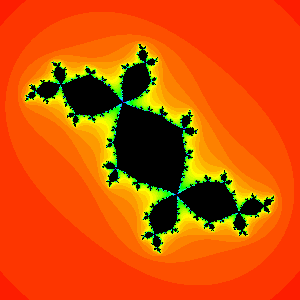Figure 13: the fractal rabbit.

Another way to say this is that the filled Julia set contains infinitely many "junction points" at which 3 distinct black regions in Jc are attached. In figure 14 we have magnified a portion of the fractal rabbit to illustrate this.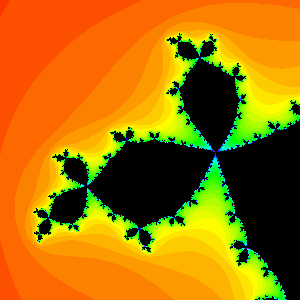Figure 14: a magnification of the fractal rabbit.

The fact that each junction point in this filled Julia set has 3 pieces attached is no surprise to those in the know, since this c-value lies in a primary period 3 bulb in the Mandelbrot set. This is another fascinating fact about M. If you choose a c-value from one of the primary decorations in M, then, first of all, Jc must be a connected set, and secondly, Jc contains infinitely many special junction points and each of these points has exactly n regions attached to it, where n is exactly the period of the bulb. Figure 15 illustrates this for periods 4 and 5.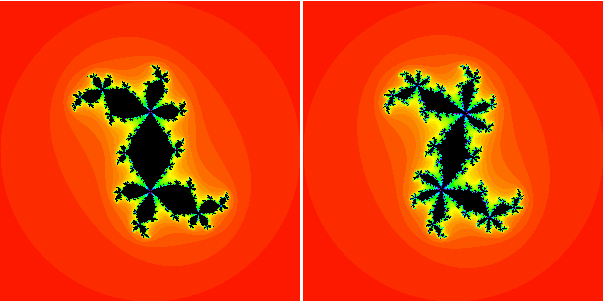Figure 15: period 4 and period 5 filled Julia sets.

My website has a collection of movies showing how the Julia set changes and how the junction points arise as the c-value moves from the main cardioid of the Mandelbrot set into the primary bulbs.

### Summary

Thus, we've seen that the Mandelbrot set possesses an extraordinary amount of structure. We can use the geometry of M to understand the dynamics of x2 + c. Or we can take dynamical information and use it to understand the shape of M. This interplay between dynamics and geometry is on the one hand fascinating and, on the other, still not completely understood. Much of this interplay has been catalogued in recent years by mathematicians such as Adrien Douady, John H Hubbard, Jean-Christophe Yoccoz, Curt McMullen, and others, but much more remains to be discovered. If you enjoyed this article, then maybe you can continue studying the Mandelbrot set and become one of the people making the discoveries.